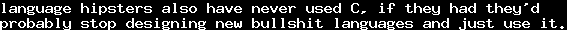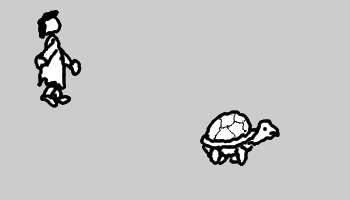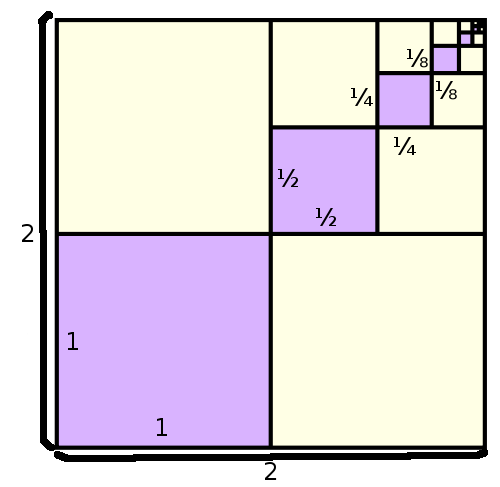# On Infinity 2 - Limit PointsSun, 25 Dec 2011 23:30:00 GMT

This is the second post of my series on infinity, be sure to read the first one.

We start this chapter with a nice story, "Achilles and the Tortoise", one of Zeno's Paradoxes, though we slightly adapt it. So, Achilles races against a tortoise. He gives the tortoise a 1 mile head start. Assuming Achilles is four times as fast as the tortoise, when he arrives 1 mile, the tortoise is at 1.25 miles. When he arrives 1.25, the tortoise is at 1.3125 miles. When he arrives at 1.3125, the tortoise is at 1.328125. And so on. Whenever Achilles reaches the point the tortoise was when he started, the tortoise already went further. This way, Achilles would have to go through infinitely many points, before reaching the tortoise.

Of course, this is not really a paradoxon. The problem lies in the fact that we have an infinitary process in here. As mathematicians do, we write the problem down more formally. Letbe the-th point where Achilles stands, andthe point where the tortoise stands. We haveand, respectively. By definition, we have, because Achilles always reaches the former point of the turtle. As the turtle has a 1 mile head start, and hastimes of Achilles' speed, we set, which is. It is easy to see, that, that is,, which is a shorter mathematical notation for such sums.

Using the axioms we saw in Part 1, we can prove this formally (if you are already a little confused, just skip this proof, it is not necessary for the further understanding): Assume there is ansuch that, then there is a smallest such. Clearly,, so. Therefore, it has a predecessor, for which we must have, sincewas minimal. But then,. Contradiction.

Obviously, Achilles will pass the tortoise at some point, and obviously, this point is greater than all. We want to find out more about this point where he passes the tortoise.The point we are looking for, is. In fact, this is provable. But it is a bit harder than the above induction.  What we have here, is a so-called geometric series. As we have, we have, that is,anddiffer in the termsand, and thus we haveFrom this, we see that, the largergets, the smallergets, and therefore, for very large,approaches. In fact, if we did a little more preliminary work, this would be a valid mathematical proof, and mathematicians would write, whereis the symbol for "infinity", and callthe limit of this series. As we wrotefor the finite sum, we can also writefor its limit. The following graphic gives a certain geometric intuition of this fact:(source)

Of course, it is not always that easy, not every infinite process has a finite outcome. For example, obviously, the infinite sumdoes not. However, we know that its finite parts get arbitrarily large, so we might say that. This series diverges, while the above geometric series converges.

But even worse, consider the series. Its finite parts are eitheror. But you cannot find any "tendency" on what happens during infinty. This series neither converges nor diverges.is not well-defined.

The above example with the tortoise was one special geometric series, the general (infinite) geometric series is, which converges for, and then has the limit- in the case, we had, for a general proof, consider the Wikipedia-article, or any good introduction to calculus.

Even more general, we have. With this formula, we can prove a fact quite a lot of people are not willing to understand:, wheremeans the zero with infinitely many nine-digits after the decimal point. But in fact, we have, which is, which is, according to our formula,.

There is a general law about recurring decimal numbers you may know from school, namely,. For example, from this follows the above,. More precisely written, we have, which follows by our above formula by.

As you see, studying this kind of objects can get very complicated, but produces a lot of interesting outcomes. If you did not quite get the last part, do not worry, it is rather sophisticated for a non-mathematician.